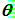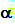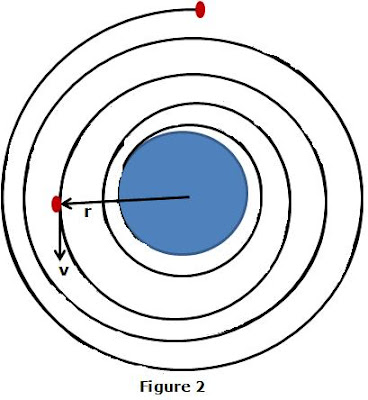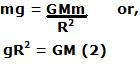## Monday, October 6, 2008

### Irodov Problem 1.227Let us first understand whats happening. Initially the satellite was in a stable orbit and the sum of its kinetic energy and its gravitational potential energy was always conserved. Then cosmic dust showed up. The cosmic dust started acting like friction, draining the energy of the satellite by trying to slow it down. When the satellite is stable its centrifugal force (as seen from the point of view of the satellite) exactly balances the gravitational attractive force due to moon. However, as the satellite slows down by a small amount, the centrifugal force falls a little short of balancing the moon gravitational force and as a consequence the satellite is pulled a bit closer to the moon. As this process continues the satellite eventually falls on to the moon. The top figure beside shows the typical scenario.

Suppose that the tangential velocity of the satellite at some given time is given by v. Let the mass of the satellite be m, and that of moon be M. Let the radius of the moon be R. Then the force due to cosmic dust acts exactly opposite to the direction of the motion (in the tangential direction) and is given by. The gravitational force continues to acts radially inwards and is given byas shown in the top figure. Since the satellite spirals inwards, the satellite does not follow a circular path and so the radius vector r and the tangential direction v are not perpendicular. Suppose that the angle between the radius vector r and the tangential velocity v isas shown in the figure.

So for an exact solution for the problem we would have to use Newton's laws. Let us consider the radial direction first. There are two forces acting in the radial direction, i) the force of gravity and ii) the component of the resistive force due to cosmic dust. In the directional perpendicular to the radius vector there is only one force acting on the satellite - the component of the resistive force due to cosmic dust. So we have,Now these equations are not trivial to solve. However, if the resistance due to the cosmic dust is very small in other wordsis very small the satellite will descend towards the moon very slowly in almost circular orbits as shown in figure 2.For this scenario, it is much easier to find an exact solution. Let the acceleration due to moon's gravity at the surface of the moon be g, then we have,If the body moves is approximately a circular orbit, the resistive force due to cosmic dust will be almost perpendicular to the radius vector. So considering the forces in the radial direction we have,At any instant of time the total energy of the satellite is given by,Since the entire energy lost by the satellite is due to the resistance due to cosmic dust, the work done by cosmic dust is equal to the loss of energy at any instant. So we have,Using (3),(6) and (7) we have,